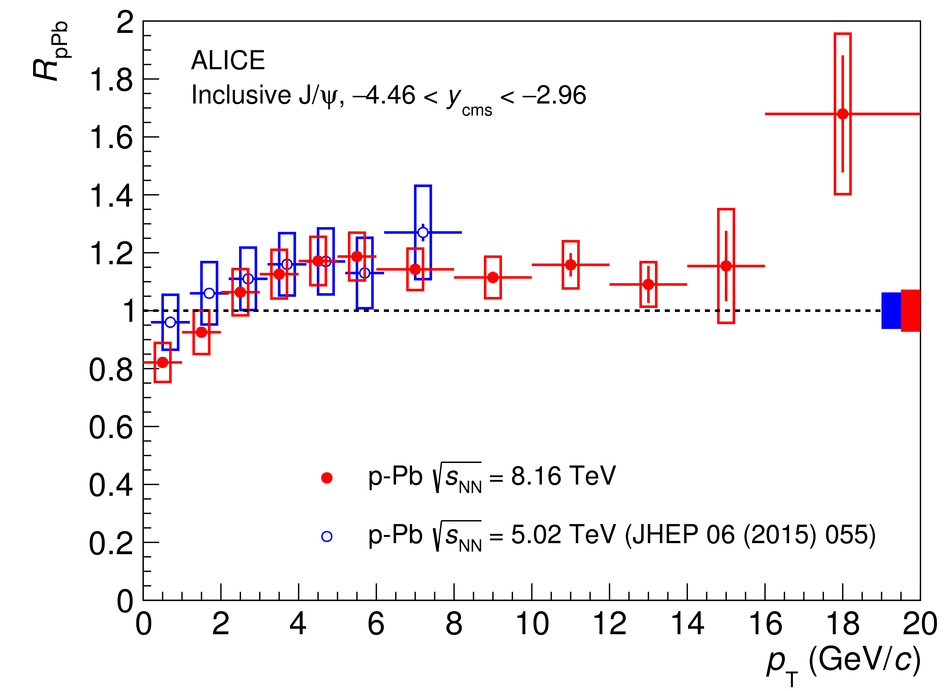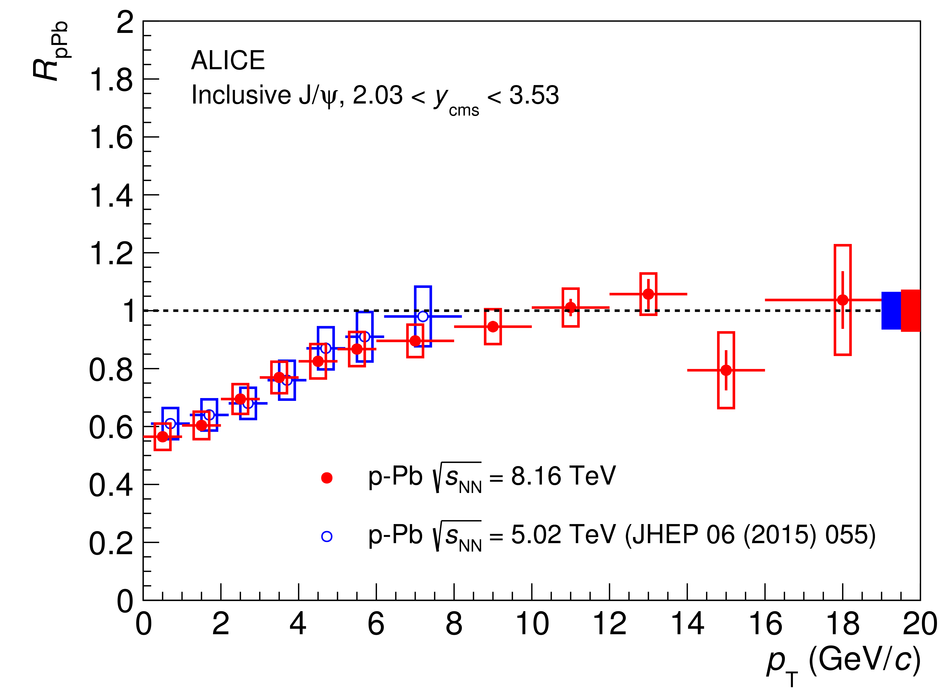# Figure 5

 The inclusive J/$\psi$ nuclear modification factor in \mbox{Pb--p} (left) and \mbox{p--Pb} (right) collisions at $\sqrt{s_{\rm NN}}=8.16$ TeV, as a function of $p_{\rm T}$. The horizontal bars correspond to the bin size. The vertical error bars represent the statistical uncertainties, the boxes around the points the uncorrelated systematic uncertainties. Correlated uncertainties are shown as a filled box around unity for each energy. The results are compared with those for \mbox{p--Pb} and \mbox{Pb--p} collisions at $\sqrt{s_{\rm NN}}=5.02$ TeV~. The latter have been plotted at a slightly shifted $p_{\rm T}$, for better visibility.